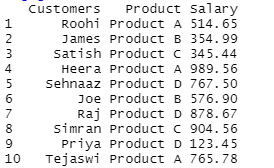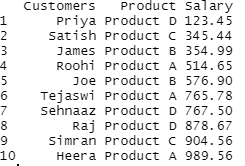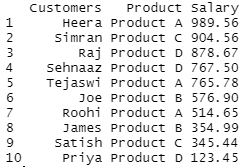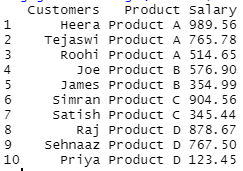Related Articles

# Sorting DataFrame in R using Dplyr

• Last Updated : 28 Jul, 2021

In this article, we will discuss about how to sort a dataframe in R programming language using Dplyr package. The package Dplyr in R programming language provides a function called arrange() function which is useful for sorting the dataframe.

Syntax :

arrange(.data, …)

The methods given below show how this function can be used in various ways to sort a dataframe.

## Sorting in Ascending order

Sorting in ascending order is the default sorting order in arrange() function. The attribute to sort by should be given as an argument to this function.

Example: Sorting the dataframe in ascending order

## R

 `# Installing the loading the package``install.packages``(``"dplyr"``)`` ` `library``(dplyr)`` ` `# Creating dataframe``gfg = ``data.frame``(Customers = ``c``(``"Roohi"``, ``"James"``, ``"Satish"``, ``"Heera"``, ``                               ``"Sehnaaz"``, ``"Joe"``,``"Raj"``, ``"Simran"``, ``                               ``"Priya"``,``"Tejaswi"``),``                  ` `                 ``Product = ``c``(``"Product A"``, ``"Product B"``, ``"Product C"``,``                             ``"Product A"``, ``"Product D"``, ``"Product B"``,``                             ``"Product D"``, ``"Product C"``, ``"Product D"``,``                             ``"Product A"``),``                  ` `                 ``Salary = ``c``(514.65, 354.99, 345.44, 989.56, 767.50,``                            ``576.90, 878.67, 904.56,123.45, 765.78)``                ``)`` ` `gfg                `` ` `# Sorting the dataframe in ascending order``arrange``(gfg, Salary)`

Output :## Sorting in Descending order

For sorting our dataframe in descending order, we will use desc() function along with the arrange() function. We will also use % operator for comparison of the dataframe column which we are taking for sorting purpose.

Example: Sorting the dataframe in descending order

## R

 `library``(dplyr)`` ` `# Creating dataframe``gfg = ``data.frame``(Customers = ``c``(``"Roohi"``, ``"James"``, ``"Satish"``, ``"Heera"``,``                               ``"Sehnaaz"``, ``"Joe"``, ``"Raj"``, ``"Simran"``, ``                               ``"Priya"``, ``"Tejaswi"``),``                  ` `                 ``Product = ``c``(``"Product A"``, ``"Product B"``, ``"Product C"``,``                             ``"Product A"``, ``"Product D"``, ``"Product B"``,``                             ``"Product D"``, ``"Product C"``, ``"Product D"``,``                             ``"Product A"``),``                  ` `                 ``Salary = ``c``(514.65, 354.99, 345.44, 989.56, 767.50, ``                            ``576.90, 878.67, 904.56,123.45, 765.78))               `` ` `# Sorting the dataframe in descending ``# order``gfg %>% ``arrange``(``desc``(Salary))`

Output :## Sorting the dataframe using multiple variables

We will now sort our dataframe using multiple variables using the arrange() function. The attributes should be given to the function separated by a column. For example, in the given example the dataframe is sorted by salary column in descending order and product column in ascending order. We will use the % operator for comparing data to be sorted in descending order.

Example: Sorting the dataframe using multiple variables

## R

 `library``(dplyr)`` ` `# Creating dataframe``gfg = ``data.frame``(Customers = ``c``(``"Roohi"``, ``"James"``, ``"Satish"``, ``"Heera"``,``                               ``"Sehnaaz"``, ``"Joe"``, ``"Raj"``, ``"Simran"``, ``                               ``"Priya"``, ``"Tejaswi"``),``                  ` `                 ``Product = ``c``(``"Product A"``, ``"Product B"``, ``"Product C"``,``                             ``"Product A"``, ``"Product D"``, ``"Product B"``,``                             ``"Product D"``, ``"Product C"``, ``"Product D"``, ``                             ``"Product A"``),``                  ` `                 ``Salary = ``c``(514.65, 354.99, 345.44, 989.56, 767.50, ``                            ``576.90, 878.67, 904.56,123.45, 765.78))``                ` `# Sorting the dataframe using ``# multiple variables``gfg %>% ``arrange``(Product, ``desc``(Salary))`

Output :My Personal Notes arrow_drop_up